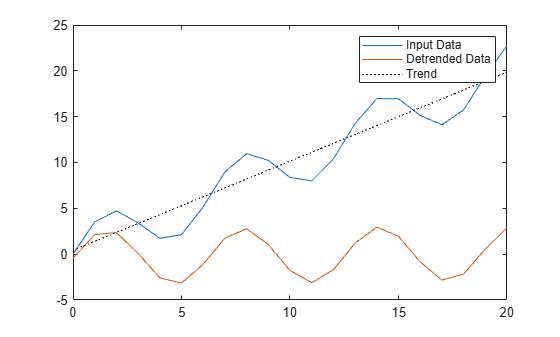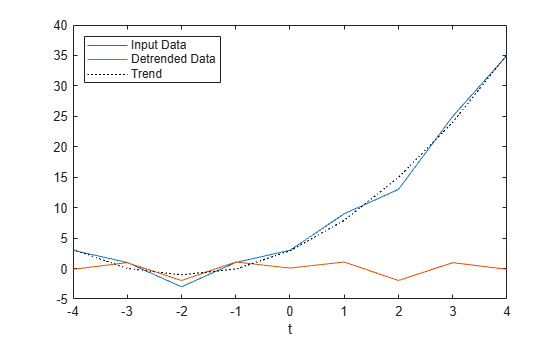# detrend

Remove polynomial trend

## Syntax

``y = detrend(x)``
``y = detrend(x,n)``
``y = detrend(x,n,bp)``
``y = detrend(___,nanflag)``
``y = detrend(___,Name,Value)``

## Description

example

````y = detrend(x)` removes the best straight-fit line from the data in `x`. If `x` is a vector, then `detrend` subtracts the trend from the elements of `x`.If `x` is a matrix, then `detrend` operates on each column separately, subtracting each trend from the corresponding column of `x`. ```

example

````y = detrend(x,n)` removes the `n`th-degree polynomial trend. For example, when ```n = 0```, `detrend` removes the mean value from `x`. When `n = 1`, `detrend` removes the linear trend, which is equivalent to the previous syntax. When `n = 2`, `detrend` removes the quadratic trend.```

example

````y = detrend(x,n,bp)` removes a continuous, piecewise trend with segments defined by the break points `bp`.```
````y = detrend(___,nanflag)` specifies how `NaN` values are treated for any of the previous syntaxes. For example, `detrend(x,'omitnan')` removes `NaN` values before calculating the trend, while `detrend(x,'includenan')` includes them (default).```

example

````y = detrend(___,Name,Value)` specifies additional parameters using one or more name-value pairs. For example, `detrend(x,1,bp,'Continuous',false)` specifies that the fitted trend can have discontinuities.```

## Examples

collapse all

Create a vector of data, and remove the continuous linear trend. Plot the original data, the detrended data, and the linear trend.

```t = 0:20; x = 3*sin(t) + t; y = detrend(x); plot(t,x,t,y,t,x-y,':k') legend('Input Data','Detrended Data','Trend','Location','northwest') ```Create a vector of data, and remove the continuous quadratic trend. Plot the original data, the detrended data, and the trend.

```t = 0:20; x = 20*sin(t) + t.^2; y = detrend(x,2); plot(t,x,t,y,t,x-y,':k') legend('Input Data','Detrended Data','Trend','Location','northwest') ```Create a vector of data, and remove the piecewise linear trend using a break point at 0. Specify that the resulting output can be discontinuous. Plot the original data, the detrended data, and the trend.

```t = -10:10; x = t.^3 + 6*t.^2 + 4*t + 3; bp = 0; y = detrend(x,1,bp,'SamplePoints',t,'Continuous',false); plot(t,x,t,y,t,x-y,':k') legend('Input Data','Detrended Data','Trend','Location','northwest') ```## Input Arguments

collapse all

Input array, specified as a vector, matrix, or multidimensional array. When `x` is a multidimensional array, `detrend` operates column-wise across all dimensions.

Data Types: `double` | `single`
Complex Number Support: Yes

Polynomial degree, specified as a non-negative integer scalar, or as `'constant'` (equivalent to `0`) or `'linear'` (equivalent to `1`).

Break points to define piecewise segments of the data, specified as a vector containing one of the following:

• Sample point values indicating the location of the break points. Sample point values are contained either in the default sample points vector ```[1 2 3 ...]``` or in the vector specified by the `'SamplePoints'` parameter.

• Logical values where logical 1 (`true`) indicates a break point in the corresponding element of the input data. If `bp` contains logical values, it must be the same length as the sample points.

Break points are useful when you want to compute separate trends for different segments of the data.

Data Types: `double` | `single` | `datetime` | `duration` | `logical`

`NaN` condition, specified as one of the following values:

• `'includenan'` — Include `NaN` values in the input data when computing the trend.

• `'omitnan'` — Ignore all `NaN` values in the input when computing the trend.

### Name-Value Arguments

Specify optional pairs of arguments as `Name1=Value1,...,NameN=ValueN`, where `Name` is the argument name and `Value` is the corresponding value. Name-value arguments must appear after other arguments, but the order of the pairs does not matter.

Before R2021a, use commas to separate each name and value, and enclose `Name` in quotes.

Example: `y = detrend(x,'SamplePoints',1:10:1000)`

Continuity constraint, specified as the comma-separated pair consisting of `'Continuous'` and one of the following:

• `true` — The fitted trend must be continuous everywhere.

• `false` — The fitted trend can contain discontinuities.

Sample points, specified as the comma-separated pair consisting of `'SamplePoints'` and a vector. The sample points represent the locations of the input data on the x-axis, and they must be unique and sorted.

Data Types: `double` | `single` | `datetime` | `duration`

## Version History

Introduced before R2006a